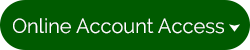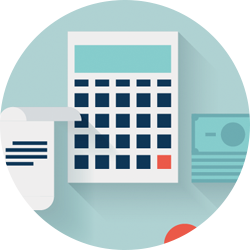Need to know how much home you can afford, how much your auto payments will be, or even how long it will take to become a millionaire?

### Auto Calculators

How much will my monthly payments be? Calculate How much can I afford for a vehicle? Calculate Which is the best vehicle loan? Calculate Should I take the rebate or special financing? Calculate Should I pay cash or finance? Calculate

### Loan Calculators

How can I pay off my debt? Calculate How much will my payments be? Calculate What if I made bi-weekly payments? Calculate

### Savings Calculators

What will my savings be worth? Calculate How long will it take for my savings goals to be met? Calculate What will it take to save for a college education? Calculate What will it take to become a millionaire? Calculate What is the cost of waiting to save? Calculate How much more can I save by spending less? Calculate How will different interest rates affect savings? Calculate

### Mortgage Calculators

What will my monthly mortgage payment be? Calculate Should I refinance? Calculate

### Debt Calculators

What is my debt ratio? Calculate Should I consolidate my debt? Calculate

### Retirement Calculators

Am I saving enough for retirement? Calculate How does an IRA, Roth IRA, and Traditional Savings compare? Calculate